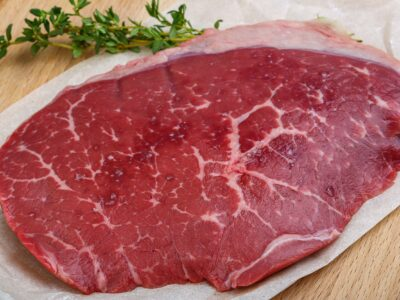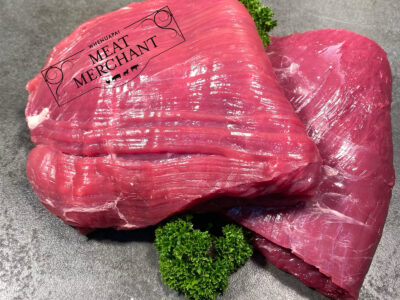Chef Rib Roast Beef\$55 jQuery(function(\$){ var price = '55'; currency = '\$'; /* \$('[name=quantity]').change(function(){ if (!(this.value < 1)) { var product_total = parseFloat(price * this.value); \$(this).closest('.woocom-list-content').find('.product_total_price .price .woocommerce-Price-amount').html(currency + product_total.toFixed(2)); } }); jQuery( '.variations_form' ).each( function() { jQuery(this).on( 'found_variation', function( event, variation ) { var price = variation.display_price; var quantity = \$(this).find('[name=quantity]').val(); if (!(quantity < 1)) { console.log(price); var product_total = parseFloat(price * quantity); \$(this).closest('.woocom-list-content').find('.product_total_price .price .woocommerce-Price-amount').html(currency + product_total.toFixed(2)); } }); });*/ }); Scotch Fillet on the bone, Rib Roast, Standing beef Roast= Devine 2 Bone Roast3 Bone Roast Chef Rib Roast quantity ADDChuck Steak Beef\$11.50 jQuery(function(\$){ var price = '11.50'; currency = '\$'; /* \$('[name=quantity]').change(function(){ if (!(this.value < 1)) { var product_total = parseFloat(price * this.value); \$(this).closest('.woocom-list-content').find('.product_total_price .price .woocommerce-Price-amount').html(currency + product_total.toFixed(2)); } }); jQuery( '.variations_form' ).each( function() { jQuery(this).on( 'found_variation', function( event, variation ) { var price = variation.display_price; var quantity = \$(this).find('[name=quantity]').val(); if (!(quantity < 1)) { console.log(price); var product_total = parseFloat(price * quantity); \$(this).closest('.woocom-list-content').find('.product_total_price .price .woocommerce-Price-amount').html(currency + product_total.toFixed(2)); } }); });*/ }); 500g1 KG Chuck Steak quantity ADDCorned Beef Beef\$20 jQuery(function(\$){ var price = '20'; currency = '\$'; /* \$('[name=quantity]').change(function(){ if (!(this.value < 1)) { var product_total = parseFloat(price * this.value); \$(this).closest('.woocom-list-content').find('.product_total_price .price .woocommerce-Price-amount').html(currency + product_total.toFixed(2)); } }); jQuery( '.variations_form' ).each( function() { jQuery(this).on( 'found_variation', function( event, variation ) { var price = variation.display_price; var quantity = \$(this).find('[name=quantity]').val(); if (!(quantity < 1)) { console.log(price); var product_total = parseFloat(price * quantity); \$(this).closest('.woocom-list-content').find('.product_total_price .price .woocommerce-Price-amount').html(currency + product_total.toFixed(2)); } }); });*/ }); Corned Beef made in store with our very own brine recipe 1 KG1.5 KG2 KG Corned Beef quantity ADDDiced Stewing Beef Beef\$10.50 jQuery(function(\$){ var price = '10.50'; currency = '\$'; /* \$('[name=quantity]').change(function(){ if (!(this.value < 1)) { var product_total = parseFloat(price * this.value); \$(this).closest('.woocom-list-content').find('.product_total_price .price .woocommerce-Price-amount').html(currency + product_total.toFixed(2)); } }); jQuery( '.variations_form' ).each( function() { jQuery(this).on( 'found_variation', function( event, variation ) { var price = variation.display_price; var quantity = \$(this).find('[name=quantity]').val(); if (!(quantity < 1)) { console.log(price); var product_total = parseFloat(price * quantity); \$(this).closest('.woocom-list-content').find('.product_total_price .price .woocommerce-Price-amount').html(currency + product_total.toFixed(2)); } }); });*/ }); Lean beef ideal for stews and casseroles, slow cookers and pressure cookers. Diced for your convenience. 500g1 KG Diced Stewing Beef quantity ADDFlank Steak Beef\$15 jQuery(function(\$){ var price = '15'; currency = '\$'; /* \$('[name=quantity]').change(function(){ if (!(this.value < 1)) { var product_total = parseFloat(price * this.value); \$(this).closest('.woocom-list-content').find('.product_total_price .price .woocommerce-Price-amount').html(currency + product_total.toFixed(2)); } }); jQuery( '.variations_form' ).each( function() { jQuery(this).on( 'found_variation', function( event, variation ) { var price = variation.display_price; var quantity = \$(this).find('[name=quantity]').val(); if (!(quantity < 1)) { console.log(price); var product_total = parseFloat(price * quantity); \$(this).closest('.woocom-list-content').find('.product_total_price .price .woocommerce-Price-amount').html(currency + product_total.toFixed(2)); } }); });*/ }); New Zealands Skirt Steak, Americans Flank Steak, Frances Bavette, Brazils Fraldinha, Colombias Sobrebarringa. What ever name you like to use, this steak is equally diverse in the many dishes it provide 500g Piece Flank Steak quantity ADDRolled Beef Beef\$20 jQuery(function(\$){ var price = '20'; currency = '\$'; /* \$('[name=quantity]').change(function(){ if (!(this.value < 1)) { var product_total = parseFloat(price * this.value); \$(this).closest('.woocom-list-content').find('.product_total_price .price .woocommerce-Price-amount').html(currency + product_total.toFixed(2)); } }); jQuery( '.variations_form' ).each( function() { jQuery(this).on( 'found_variation', function( event, variation ) { var price = variation.display_price; var quantity = \$(this).find('[name=quantity]').val(); if (!(quantity < 1)) { console.log(price); var product_total = parseFloat(price * quantity); \$(this).closest('.woocom-list-content').find('.product_total_price .price .woocommerce-Price-amount').html(currency + product_total.toFixed(2)); } }); });*/ }); Ideal for slow roasting and slow cooker 1 KG1.5 KG2 KG2.5 KG3 KG Rolled Beef quantity ADDRump Steak Beef\$13 jQuery(function(\$){ var price = '13'; currency = '\$'; /* \$('[name=quantity]').change(function(){ if (!(this.value < 1)) { var product_total = parseFloat(price * this.value); \$(this).closest('.woocom-list-content').find('.product_total_price .price .woocommerce-Price-amount').html(currency + product_total.toFixed(2)); } }); jQuery( '.variations_form' ).each( function() { jQuery(this).on( 'found_variation', function( event, variation ) { var price = variation.display_price; var quantity = \$(this).find('[name=quantity]').val(); if (!(quantity < 1)) { console.log(price); var product_total = parseFloat(price * quantity); \$(this).closest('.woocom-list-content').find('.product_total_price .price .woocommerce-Price-amount').html(currency + product_total.toFixed(2)); } }); });*/ }); Under rated frying steak, most flavoursome of all the tradition tender cuts. Speak to the team to discover their tips on getting a succulent juicy result 400g500g800g1 KG Rump Steak quantity ADDSirloin Steak Beef\$10 jQuery(function(\$){ var price = '10'; currency = '\$'; /* \$('[name=quantity]').change(function(){ if (!(this.value < 1)) { var product_total = parseFloat(price * this.value); \$(this).closest('.woocom-list-content').find('.product_total_price .price .woocommerce-Price-amount').html(currency + product_total.toFixed(2)); } }); jQuery( '.variations_form' ).each( function() { jQuery(this).on( 'found_variation', function( event, variation ) { var price = variation.display_price; var quantity = \$(this).find('[name=quantity]').val(); if (!(quantity < 1)) { console.log(price); var product_total = parseFloat(price * quantity); \$(this).closest('.woocom-list-content').find('.product_total_price .price .woocommerce-Price-amount').html(currency + product_total.toFixed(2)); } }); });*/ }); Sirloin Steak aka: Porterhouse Steak. Equally as tender as scotch fillet, external fat, ideal for crispy fat (250g-280g) 1.5kg Piece1Kg Steaks2.5kg PieceOne Steak (250g ish)1 KG Piece Sirloin Steak quantity ADDTasty Italian Meatballs Beef\$10 jQuery(function(\$){ var price = '10'; currency = '\$'; /* \$('[name=quantity]').change(function(){ if (!(this.value < 1)) { var product_total = parseFloat(price * this.value); \$(this).closest('.woocom-list-content').find('.product_total_price .price .woocommerce-Price-amount').html(currency + product_total.toFixed(2)); } }); jQuery( '.variations_form' ).each( function() { jQuery(this).on( 'found_variation', function( event, variation ) { var price = variation.display_price; var quantity = \$(this).find('[name=quantity]').val(); if (!(quantity < 1)) { console.log(price); var product_total = parseFloat(price * quantity); \$(this).closest('.woocom-list-content').find('.product_total_price .price .woocommerce-Price-amount').html(currency + product_total.toFixed(2)); } }); });*/ }); Handcrafted in store from our very own recipe. Beef mince with beef sausage meat and a touch of herbs served with our in house Italiano Sauce. Quick and easy meal, just like you made it yourself. 6 Pack8 Pack10 Pack12 Pack Tasty Italian Meatballs quantity ADD